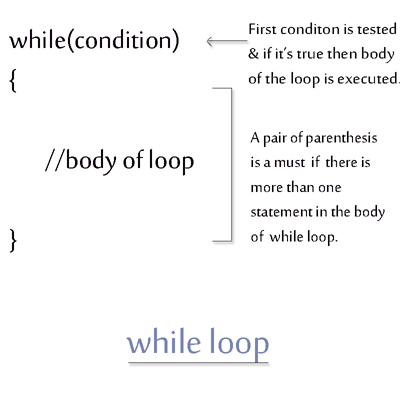< Prev
Next >

# C++ While Loop

C++ provides another decision-making looping structure known as while loop, in which the condition of while loop is tested for the boolean value - true or false.
• If the condition is true then the block of statements in while loop are executed.
• If the condition is false then the block of statements in the while loop are skipped and not executed.## while loop example

``````// C++ while loop example

#include<iostream>

using namespace std;
int main() // main method starts
{

int i=0;

while(i<3)
{
cout<<"i = " << i <<"\n";
i++;
}

return 0;
}   // main method ends and program ends too
``````

## Output

``````i = 0
i = 1
i = 2``````

## While loop must have an iteration part in its body or it runs an endless loop

``````// Endless while loop

#include<iostream>

using namespace std;
int main() // main method starts
{

int i=0;

while(i<3)
{
cout<<"i = "<< i <<"\n";
}

return 0;
}  /*main method and program ends here */
``````

## Output

This program when executed, runs indefinitely in a loop because of the absence of incremental part to increment the value of i.

< Prev
Next >
< Do-While Loop
Break Statement >